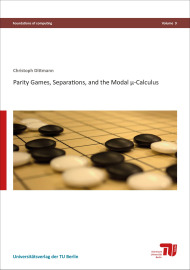# Parity games, separations, and the modal μ-calculusSize: 284 pages
Format: 14,8 x 21,0 cm
Publishing year: 2017
ISBN 978-3-7983-2887-7
13,00

The topics of this thesis are the modal μ-calculus and parity games. The modal μ-calculus is a common logic for model-checking in computer science. The model-checking problem of the modal μ-calculus is polynomial time equivalent to solving parity games, a 2-player game on labeled directed graphs. We present the first FPT algorithms (fixed-parameter tractable) for the model-checking problem of the modal μ-calculus on restricted classes of graphs, specifically on classes of bounded Kelly-width or bounded DAG-width. In this process we also prove a general decomposition theorem for the modal μ-calculus and define a useful notion of type for this logic. Then, assuming a class of parity games has a polynomial time algorithm solving it, we consider the problem of extending this algorithm to larger classes of parity games. In particular, we show that joining games, pasting games, or adding single vertices preserves polynomial-time solvability. It follows that parity games can be solved in polynomial time if their underlying undirected graph is a tournament, a complete bipartite graph, or a block graph. In the last chapter we present the first non-trivial formal proof about parity games. We explain a formal proof of positional determinacy of parity games in the proof assistant Isabelle/HOL.